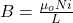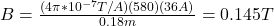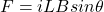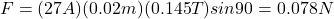## A 580-turn solenoid is 18 cm long. The current in it is 36 A. A straight wire cuts through the center of the solenoid, along a 2.0-cm diamet

Question

A 580-turn solenoid is 18 cm long. The current in it is 36 A. A straight wire cuts through the center of the solenoid, along a 2.0-cm diameter. This wire carries a 27-A current downward (and is connected by other wires that don’t concern us). What is the magnitude of the force on this wire assuming the solenoid’s field points due east?

in progress 0
5 months 2021-08-25T11:28:33+00:00 2 Answers 2 views 0

1. Answer: The magnitude of the force is 0.079N

Explanation: Please see the attachments below

F = 0.078N

Explanation:

In order to calculate the magnitude of the force on the wire you first calculate the magnitude of the magnetic field generated by the solenoid, by using the following formula:(1)

μo: magnetic permeability of vacuum = 4π*10^-7 T/A

N: turns of the solenoid = 580

i: current in the solenoid = 36A

L: length of the solenoid = 18cm = 0.18m

You replace the values of all parameters in the equation (1):Next, you calculate the force exerted on the wire, by using the following formula:(2)

i: current in the wire = 27A

L: length of the wire that perceives the magnetic field (the same as the radius of the solenoid) = 2.0 cm = 0.02m

θ: angle between wire and the direction of B

B: magneitc field in the solenoid = 0.145T

The direction of the wire are perpendicular to the direction of the magnetic field, hence, the angle is 90°.

You replace the values of the parameters in the equation (2):The magnitude of the force on the wire is 0.078N